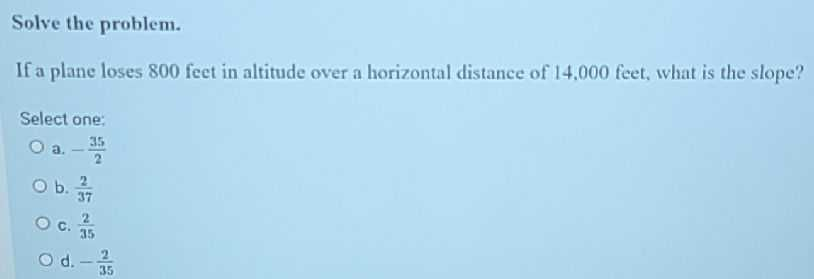### Still have math questions?

Algebra
QuestionIf a plane loses $$800$$ feet in altitude over a horizontal distance of $$14.000$$ feet, what is the slope? Select one:

a. $$- \frac { 35 } { 2 }$$

b. $$\frac { 2 } { 37 }$$

c. $$\frac { 2 } { 35 }$$

d. $$- \frac { 2 } { 35 }$$

slope = $$\frac{2}{35}$$